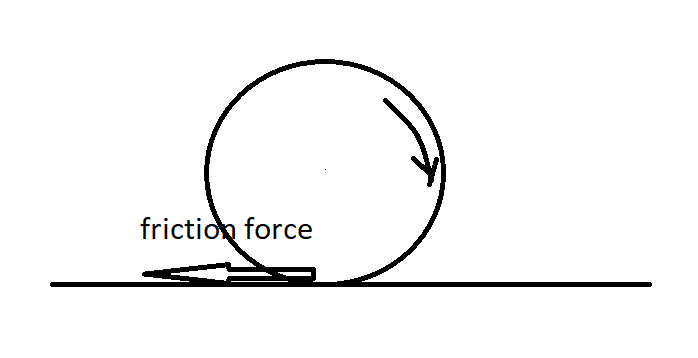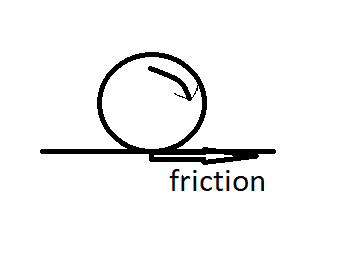# Rolling without slipping in non-sloping surface

• B
According to Newton's first law, an object left on a non-sloping surface that rolls and moves forward without slipping will continue to move indefinitely, as long as there is no external force acting on it. However, in reality, the ball will eventually slow down due to factors such as rolling resistance and air resistance. The friction force does not necessarily decrease velocity, but rather plays a role in deceleration. This is because friction is necessary for acceleration and deceleration, not for maintaining constant velocity. In the case of a rolling object, the friction force is zero and it will continue to roll uniformly on a frictionless surface. The amount of friction affects the distance traveled, with less friction resulting in longer distances. Rolling resistancef

Suppose we leave an object on a non-sloping surface that rolls and moves forward without slipping. Does this object continue to move indefinitely?

Suppose we leave an object on a non-sloping surface that rolls and moves forward without slipping. Does this object continue to move indefinitely?
Theoretically, Newton's first law applies.

but in free-body diagram, the friction force decrease velocity, isn't it?but in free-body diagram, the friction force decrease velocity, isn't it?

View attachment 299644
For rolling at constant velocity the friction force is zero. If the friction force were non-zero, then there would be a torque on the ball.

Given that the motion is rolling, it means that the friction is non-zero. Like a ball dropped on the ground.

Given that the motion is rolling, it means that the friction is non-zero.
The friction is zero. The ball would continue to roll uniformly on a frictionless surface. Friction is only required for acceleration and deceleration.

In fact, if you add some air resistance to slow the ball down, then the friction acts in the direction of motion. In the opposite direction to what you have shown.

Consider two modes, one with low friction and the other with more friction
The rolling body travels longer distances on the surface with less friction.

Consider two modes, one with low friction and the other with more friction
The rolling body travels longer distances on the surface with less friction.
It's rolling resistance, not friction, that determines the deceleration:

https://en.wikipedia.org/wiki/Rolling_resistance

The reason a wheel is so efficient is that there is effectively no friction for a uniformly rolling wheel.

but in free-body diagram, the friction force decrease velocity, isn't it?

View attachment 299644
Note that, in particular, in your model the ball will be slowing down, but its rotation will be speeding up!

This diagram is probably correct because friction reduces rolling, but it contradicts Newton's second law.This diagram is probably correct because friction reduces rolling, but it contradicts Newton's second law.
View attachment 299646
That diagram is equally wrong. Look at the Wikipedia page. Rolling resistance is complicated and cannot be reduced to a single force of friction.

This diagram is probably correct because friction reduces rolling, but it contradicts Newton's second law.
View attachment 299646
because the motion is negative acceleration not possitive acceleration

Does this object continue to move indefinitely?
In practice or in theory?
The loss of energy will be a function of the distance traveled and the airspeed.
Theoretically the ball will gradually slow down, but will never quite stop.
Practically the surface will not be perfectly smooth, so the ball will stop at the foot of a hill.

If a body rolls without slipping on a surface then the friction force even if it is not vanished does not make work